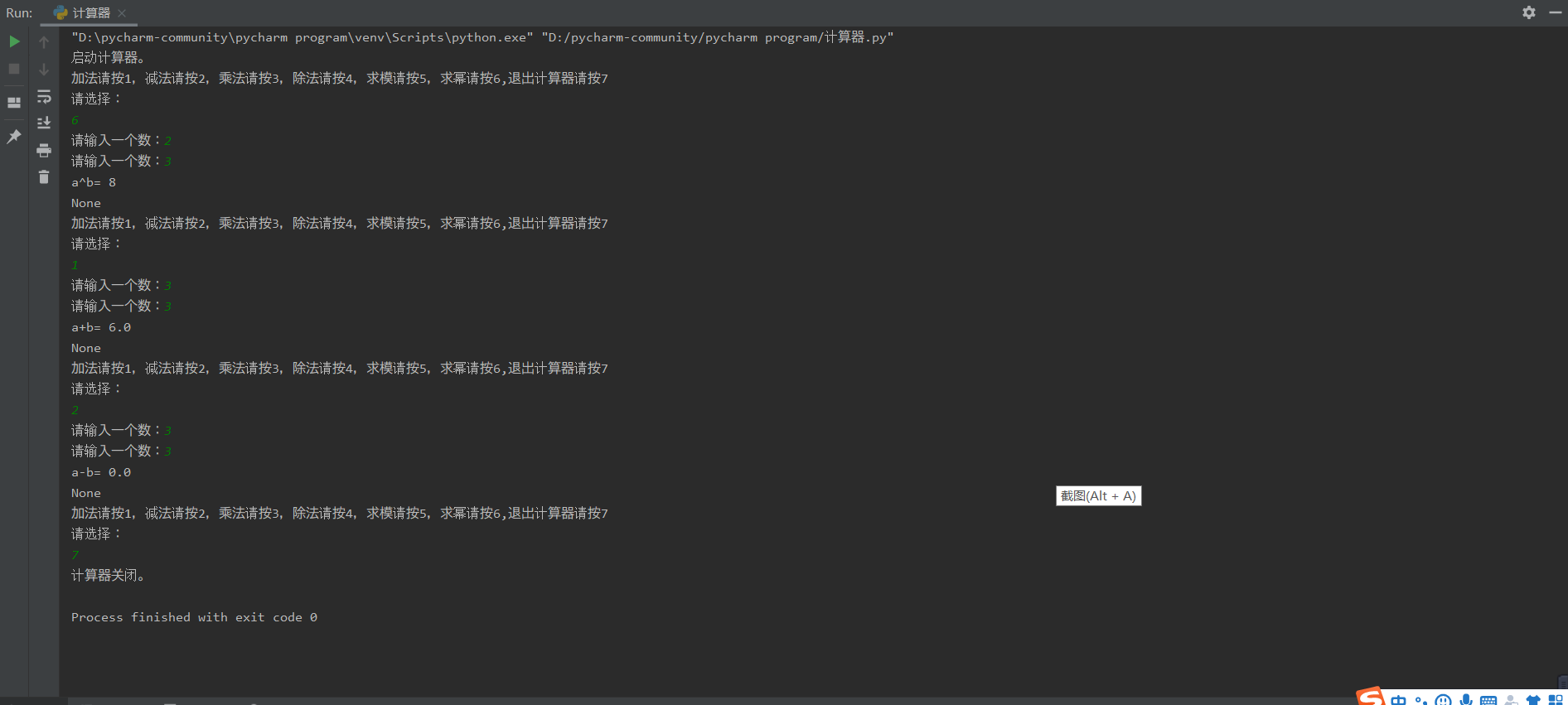# 学号 2019-2020-2 《Python程序设计》实验x报告

## 1.实验内容

·设计并完成一个完整的应用程序，完成加减乘除模等运算，功能多多益善。

·考核基本语法、判定语句、循环语句、逻辑运算等知识点

## 2. 实验过程及结果

def Sum(a,b):
print("a+b=",a+b)
def Subtraction(a,b):
print("a-b=",a-b)
def Multiplication(a,b):
print("a*b=：",a*b)
def Division(a,b):
if b != 0:
print("a/b=：",a/b)
else:
print("无法计算！")
def Mo(a,b):
print("两数求模为",a%b)
def Mifang(a,b):
print("a^b=",a**b)

print("启动计算器。")
while True:
print("加法请按1，减法请按2，乘法请按3，除法请按4，求模请按5，求幂请按6,退出计算器请按7")
print("请选择：")
x = int(input())
if x == 7:
print("计算器关闭。")
break
if x == 6:
a = int(input("请输入一个数："))
b = int(input("请输入一个数："))
print(Mifang(a,b))
a = float(input("请输入一个数："))
b = float(input("请输入一个数："))
if x == 1:
print(Sum(a,b))
elif x == 2:
print(Subtraction(a,b))
elif x == 3:
print(Multiplication(a,b))
elif x == 4:
print(Division(a,b))
elif x == 5:
print(Mo(a,b))## 3. 实验过程中遇到的问题和解决过程

• 问题1：关闭计算器时前还要输入a和b
• 问题1解决方案：将关闭计算器有关的代码前移。
• 问题2：进行幂方计算时程序错误
• 问题2解决方案：float型无法进行幂方计算，将幂方计算有关代码前移，a和b改为int型，同时加上continue函数

## 其他（感悟、思考等）

posted @ 2020-04-20 19:52  天澜(>_<)  阅读(168)  评论(0编辑  收藏